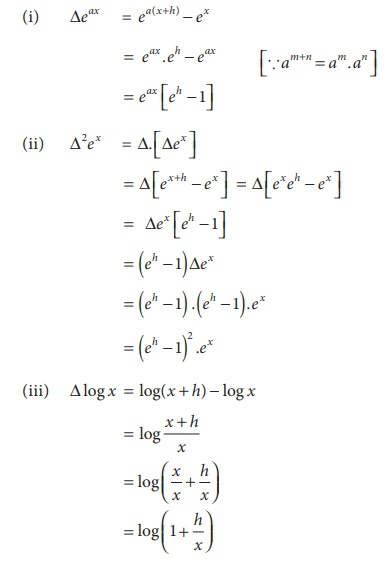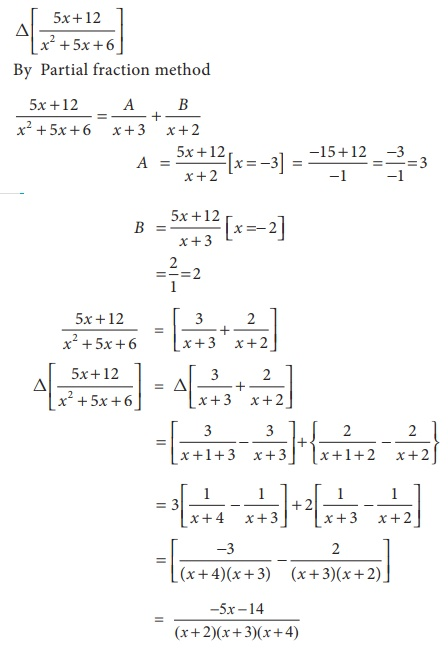Home | | Business Maths 12th Std | Finite Differences: Solved Example Problems

# Finite Differences: Solved Example Problems

Numerical Methods: Finite Differences: Solved Example Problems

Example 5.1

Construct a forward difference table for the following dataSolution:

The Forward difference table is given belowExample 5.2

Construct a forward difference table for y = f(x) = x 3 + 2x + 1 for x = 1,2,3,4,5

Solution:

y = f(x) = x3 + 2 x + 1 for x =1,2,3,4,5Example 5.3

By constructing a difference table and using the second order differences as constant, find the sixth term of the series 8,12,19,29,42ŌĆ”

Solution:

Let k be the sixth term of the series in the difference table

First we find the forward differences.Given that the second differences are constant

Ōł┤ k ŌĆō 55 = 3

k = 58

Ōł┤ the sixth term of the series is 58

Example 5.4

Find (i) Ōłåeax (ii) Ōłå2ex  (iii) Ōłålogx

Solution:Example 5.5

Evaluateby taking ŌĆś1ŌĆÖ as the interval of differencing.

Solution:Example 5.5

Evaluateby taking ŌĆś1ŌĆÖ as the interval of differencing.

Solution:Example 5.7

Prove that f(4) = f(3) + ╬öf(2) + ╬ö2f(1) + ╬ö3f(1) taking ŌĆś1ŌĆÖ as the interval of differencing.

Solution:

We know that f(4) - f(3) = ╬ö f(4)

f(4) - f(3) = ╬ö f(3)

= ╬ö [ f(2) + ╬öf(2) ]    i.e.[ f(3) - f(2) = ╬öf(2) ]

= ╬ö f(2) + ╬ö2f(2)

= ╬ö f(2) + ╬ö2[f(1)+ ╬öf(1)]

f(4) = f(3) + ╬öf(2) + ╬ö2 f(1) + ╬ö3 f(1)

Example 5.8

Given U0 =1, U1 =11, U2 =21, U3 =28 and U4 =29 find ╬ö4U0

Solution:

╬ö4U0 = (E-1) 4U0Tags : Numerical Methods , 12th Business Maths and Statistics : Chapter 5 : Numerical Methods
Study Material, Lecturing Notes, Assignment, Reference, Wiki description explanation, brief detail
12th Business Maths and Statistics : Chapter 5 : Numerical Methods : Finite Differences: Solved Example Problems | Numerical Methods